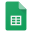Metric PrefixesMetric Prefixes

Notes:

1. The long and short scales are two of several large-number naming systems for integer powers of ten that use the same words with different meanings:
Long scale: Every new term greater than million is one million times larger than the previous term. Thus, billion means a million millions (1012), trillion means a million billions (1018), and so on.
Short scale: Every new term greater than million is one thousand times larger than the previous term. Thus, billion means a thousand millions (109), trillion means a thousand billions (1012), and so on. The short scale is typically used in the U.S.
See